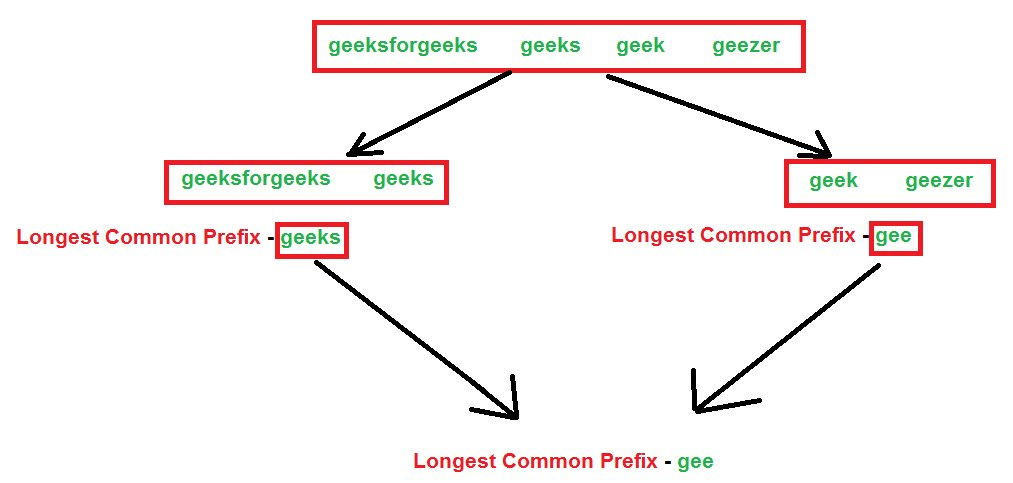# Longest Common Prefix using Divide and Conquer Algorithm

• Difficulty Level : Medium
• Last Updated : 12 Jun, 2021

Given a set of strings, find the longest common prefix.

Examples:

```Input  : {“geeksforgeeks”, “geeks”, “geek”, “geezer”}
Output : "gee"

Input  : {"apple", "ape", "april"}
Output : "ap"```

We have discussed word by word matching and character by character matching algorithms.
In this algorithm, a divide and conquer approach is discussed. We first divide the arrays of string into two parts. Then we do the same for left part and after that for the right part. We will do it until and unless all the strings become of length 1. Now after that, we will start conquering by returning the common prefix of the left and the right strings.
The algorithm will be clear using the below illustration. We consider our strings as – “geeksforgeeks”, “geeks”, “geek”, “geezer”.Below is the implementation.

## C++

 `//  A C++ Program to find the longest common prefix``#include``using` `namespace` `std;` `// A Utility Function to find the common prefix between``// strings- str1 and str2``string commonPrefixUtil(string str1, string str2)``{``    ``string result;``    ``int` `n1 = str1.length(), n2 = str2.length();` `    ``for` `(``int` `i=0, j=0; i<=n1-1&&j<=n2-1; i++,j++)``    ``{``        ``if` `(str1[i] != str2[j])``            ``break``;``        ``result.push_back(str1[i]);``    ``}``    ``return` `(result);``}` `// A Divide and Conquer based function to find the``// longest common prefix. This is similar to the``// merge sort technique``string commonPrefix(string arr[], ``int` `low, ``int` `high)``{``    ``if` `(low == high)``        ``return` `(arr[low]);` `    ``if` `(high > low)``    ``{``        ``// Same as (low + high)/2, but avoids overflow for``        ``// large low and high``        ``int` `mid = low + (high - low) / 2;` `        ``string str1 = commonPrefix(arr, low, mid);``        ``string str2 = commonPrefix(arr, mid+1, high);` `        ``return` `(commonPrefixUtil(str1, str2));``    ``}``}` `// Driver program to test above function``int` `main()``{``    ``string arr[] = {``"geeksforgeeks"``, ``"geeks"``,``                    ``"geek"``, ``"geezer"``};``    ``int` `n = ``sizeof` `(arr) / ``sizeof` `(arr);` `    ``string ans = commonPrefix(arr, 0, n-1);` `    ``if` `(ans.length())``        ``cout << ``"The longest common prefix is "``             ``<< ans;``    ``else``        ``cout << ``"There is no common prefix"``;``    ``return` `(0);``}`

## Java

 `// Java Program to find the longest common prefix` `class` `GFG {` `// A Utility Function to find the common prefix between``// strings- str1 and str2``    ``static` `String commonPrefixUtil(String str1, String str2) {``        ``String result = ``""``;``        ``int` `n1 = str1.length(), n2 = str2.length();` `        ``for` `(``int` `i = ``0``, j = ``0``; i <= n1 - ``1` `&&``                ``j <= n2 - ``1``; i++, j++) {``            ``if` `(str1.charAt(i) != str2.charAt(j)) {``                ``break``;``            ``}``            ``result += str1.charAt(i);``        ``}``        ``return` `(result);``    ``}` `// A Divide and Conquer based function to find the``// longest common prefix. This is similar to the``// merge sort technique``    ``static` `String commonPrefix(String arr[], ``int` `low, ``int` `high) {``        ``if` `(low == high) {``            ``return` `(arr[low]);``        ``}` `        ``if` `(high > low) {``            ``// Same as (low + high)/2, but avoids overflow for``            ``// large low and high``            ``int` `mid = low + (high - low) / ``2``;` `            ``String str1 = commonPrefix(arr, low, mid);``            ``String str2 = commonPrefix(arr, mid + ``1``, high);` `            ``return` `(commonPrefixUtil(str1, str2));``        ``}``        ``return` `null``;``    ``}` `// Driver program to test above function``    ``public` `static` `void` `main(String[] args) {``        ``String arr[] = {``"geeksforgeeks"``, ``"geeks"``,``            ``"geek"``, ``"geezer"``};``        ``int` `n = arr.length;` `        ``String ans = commonPrefix(arr, ``0``, n - ``1``);` `        ``if` `(ans.length() != ``0``) {``            ``System.out.println(``"The longest common prefix is "``                    ``+ ans);``        ``} ``else` `{``            ``System.out.println(``"There is no common prefix"``);``        ``}``    ``}``}``/* This JAVA code is contributed by 29AjayKumar*/`

## Python3

 `# A Python3 Program to find the longest common prefix` `# A Utility Function to find the common``# prefix between strings- str1 and str2``def` `commonPrefixUtil(str1, str2):` `    ``result ``=` `""``    ``n1, n2 ``=` `len``(str1), ``len``(str2)``    ``i, j ``=` `0``, ``0` `    ``while` `i <``=` `n1 ``-` `1` `and` `j <``=` `n2 ``-` `1``:``    ` `        ``if` `str1[i] !``=` `str2[j]:``            ``break``        ``result ``+``=` `str1[i]``        ``i, j ``=` `i ``+` `1``, j ``+` `1``    ` `    ``return` `result` `# A Divide and Conquer based function to``# find the longest common prefix. This is``# similar to the merge sort technique``def` `commonPrefix(arr, low, high):` `    ``if` `low ``=``=` `high:``        ``return` `arr[low]` `    ``if` `high > low:``    ` `        ``# Same as (low + high)/2, but avoids``        ``# overflow for large low and high``        ``mid ``=` `low ``+` `(high ``-` `low) ``/``/` `2` `        ``str1 ``=` `commonPrefix(arr, low, mid)``        ``str2 ``=` `commonPrefix(arr, mid ``+` `1``, high)` `        ``return` `commonPrefixUtil(str1, str2)` `# Driver Code``if` `__name__ ``=``=` `"__main__"``:` `    ``arr ``=` `[``"geeksforgeeks"``, ``"geeks"``,``                   ``"geek"``, ``"geezer"``]``    ``n ``=` `len``(arr)``    ``ans ``=` `commonPrefix(arr, ``0``, n ``-` `1``)` `    ``if` `len``(ans):``        ``print``(``"The longest common prefix is"``, ans)``    ``else``:``        ``print``(``"There is no common prefix"``)` `# This code is contributed by Rituraj Jain`

## C#

 `// C# Program to find the longest``// common prefix``using` `System;` `class` `GFG``{``// A Utility Function to find``// the common prefix between``// strings- str1 and str2``static` `string` `commonPrefixUtil(``string` `str1,``                               ``string` `str2)``{``    ``string` `result = ``""``;``    ``int` `n1 = str1.Length,``        ``n2 = str2.Length;` `    ``for` `(``int` `i = 0, j = 0;``             ``i <= n1 - 1 && j <= n2 - 1;``             ``i++, j++)``    ``{``        ``if` `(str1[i] != str2[j])``            ``break``;``        ``result += str1[i];``    ``}``    ``return` `(result);``}` `// A Divide and Conquer based``// function to find the longest``// common prefix. This is similar``// to the merge sort technique``static` `string` `commonPrefix(``string` `[]arr,``                           ``int` `low, ``int` `high)``{``    ``if` `(low == high)``        ``return` `(arr[low]);` `    ``if` `(high > low)``    ``{``        ``// Same as (low + high)/2,``        ``// but avoids overflow for``        ``// large low and high``        ``int` `mid = low + (high - low) / 2;` `        ``string` `str1 = commonPrefix(arr, low, mid);``        ``string` `str2 = commonPrefix(arr, mid + 1, high);` `        ``return` `(commonPrefixUtil(str1, str2));``    ``}``    ``return` `null``;``}` `// Driver Code``public` `static` `void` `Main()``{``    ``String []arr = {``"geeksforgeeks"``, ``"geeks"``,``                    ``"geek"``, ``"geezer"``};``    ``int` `n = arr.Length;` `    ``String ans = commonPrefix(arr, 0, n - 1);` `    ``if` `(ans.Length!= 0)``    ``{``        ``Console.Write(``"The longest common "` `+``                         ``"prefix is "` `+ ans);``    ``}``    ``else``    ``{``        ``Console.Write(``"There is no common prefix"``);``    ``}``}``}` `// This code is contributed by 29AjayKumar`

## Javascript

 ``

Output :

`The longest common prefix is gee`

Time Complexity: Since we are iterating through all the characters of all the strings, so we can say that the time complexity is O(N M) where,

```N = Number of strings
M = Length of the largest string string```

Auxiliary Space: To store the longest prefix string we are allocating space which is O(M Log N).

This article is contributed by Rachit Belwariar. If you like GeeksforGeeks and would like to contribute, you can also write an article and mail your article to review-team@geeksforgeeks.org. See your article appearing on the GeeksforGeeks main page and help other Geeks.